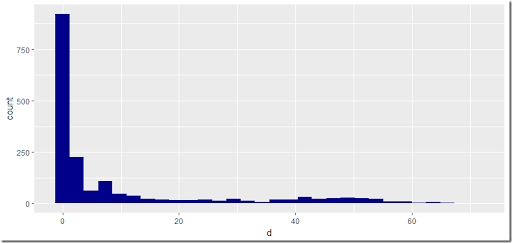## Monday, July 17, 2017

### Modeling flood damages

While working on a investment planning model to combat damages resulting from flooding, I received the results from a rainfall model that calculates damages as a result of excessive rain and flooding. The picture the engineers produced looks like:

This picture has the scenario on the x-axis (sorted by damage) and the damages on the y-axis. This picture is very much like a load-duration curve in power generation.

For a more “statistical” picture we can use standard histogram (after binning the data):##### Gamma distribution

We can use standard techniques to fit a distribution. When considering a Gamma distribution (1), a simple approach is the method of moments. The mean and the variance of the Gamma distribution with parameters $$k$$ and $$\theta$$ are given by:

 \begin{align} &E[X] = k \cdot \theta\\ & Var[X] = k \cdot \theta^2 \end{align}

Using sample mean $$\mu$$ and standard deviation $$\sigma$$, we can solve:

 \begin{align} & k \cdot \theta = \mu\\ & \sqrt{k} \cdot \theta = \sigma \end{align}

This can be easily solved numerically and it actually seems to work:

##### Weibull distribution

An alternative distribution that is sometimes suggested is the Weibull distribution (2).  The method of moments estimator for the Weibull distribution with parameters $$\lambda$$ and $$k$$ can be found by solving the system:

 \begin{align} & \lambda \Gamma(1+1/k) = \mu\\ &\lambda \sqrt{\Gamma(1+2/k)+\left(\Gamma(1+1/k)\right)^2} = \sigma \end{align}
I was unable to get a solution from this: solvers failed miserably on this.

An alternative approach would be to use an MLE (Maximum Likelihood Estimation) technique. This yields a system of equations:

 \begin{align} & \lambda^k = \frac{1}{n}\sum_{i=1}^n x_i^k\\ &k^{-1} = \frac{\sum_{i=1}^n x_i^k \ln x_i}{\sum_{i=1}^n x_i^k} – \frac{1}{n}\sum_{i=1}^n \ln x_i \end{align}
Note that we can solve the second equation first to solve for $$k$$ and then calculate $$\lambda$$ using the first equation. A solver like CONOPT will do this automatically by recognizing the triangular structure of this system. Note that our data set contains many $$x_i=0$$. These are replaced by $$x_i=0.001$$ so we can take the logarithm.

This gives is a very similar picture:

##### References
1. Gamma distribution, https://en.wikipedia.org/wiki/Gamma_distribution
2. Weibull distribution, https://en.wikipedia.org/wiki/Weibull_distribution